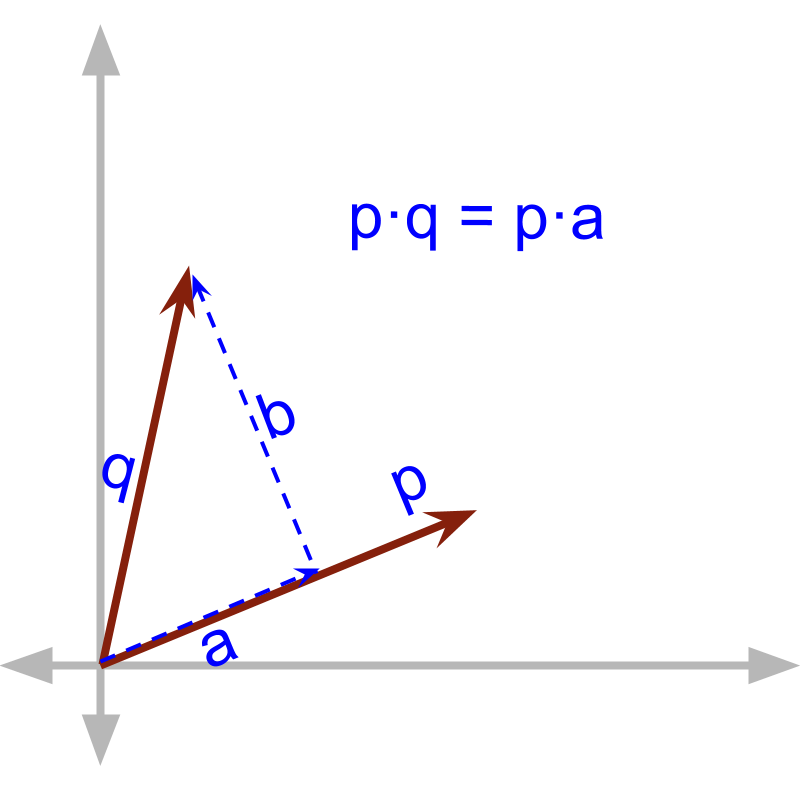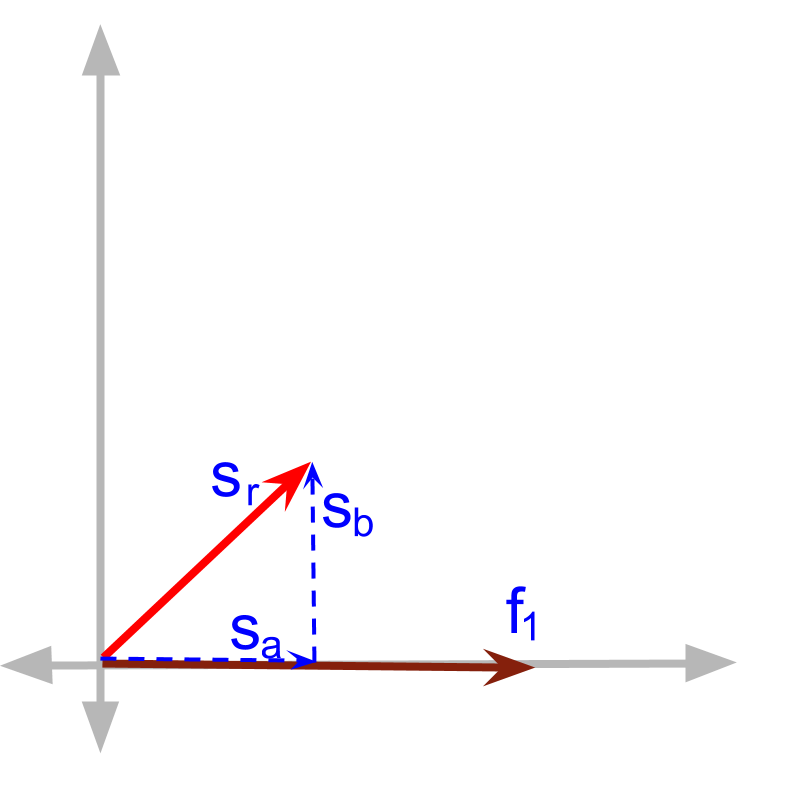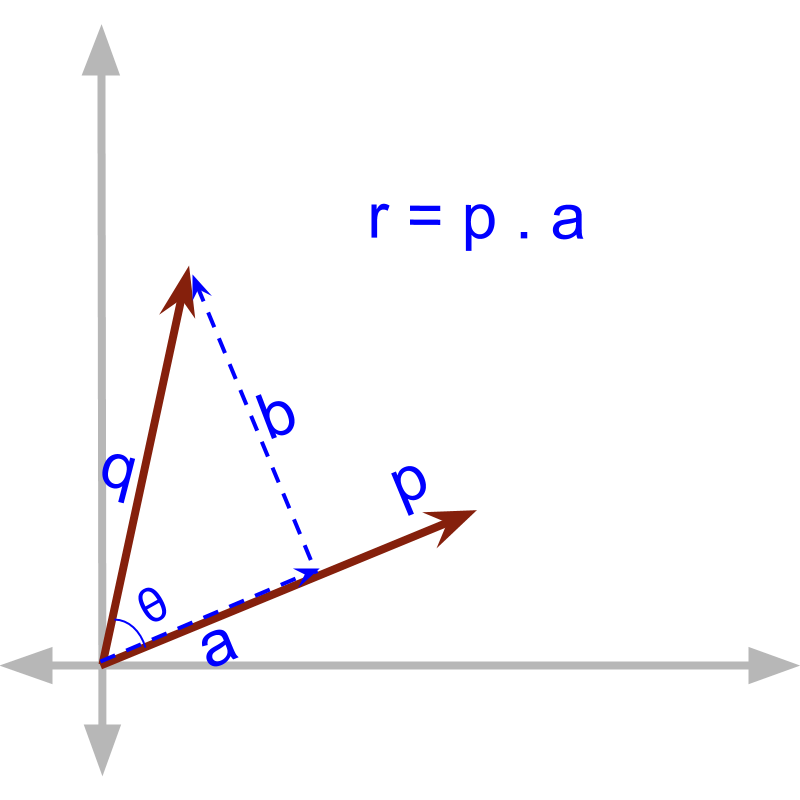maths > vector-algebra

Vector Dot Product : First Principles

what you'll learn...

Overview

Vector Dot Product: First Principles

»  multiplied with component in parallel→  $\stackrel{\to }{p}\cdot \stackrel{\to }{q}$$\vec{p} \cdot \vec{q}$ $=\stackrel{\to }{p}\cdot \stackrel{\to }{a}$$= \vec{p} \cdot \vec{a}$ $=|\stackrel{\to }{p}||\stackrel{\to }{a}|$$= | \vec{p} | | \vec{a} |$
→  product of magnitudes of components in parallel

in parallel

We have developed the understanding that
•  In some application scenarios, effect will be in the same direction as that of the cause.
•  the observed effect can be sum of the effects of multiple different causes.A vector can be split into components in parallel and in perpendicular directions to another vector.

Consider that force ${\stackrel{\to }{f}}_{1}$${\vec{f}}_{1}$ is acting on an object which moves displacement ${\stackrel{\to }{s}}_{r}$${\vec{s}}_{r}$. The effect ${\stackrel{\to }{s}}_{r}$${\vec{s}}_{r}$ is split into components ${\stackrel{\to }{s}}_{a}$${\vec{s}}_{a}$ and ${\stackrel{\to }{s}}_{b}$${\vec{s}}_{b}$, where ${\stackrel{\to }{s}}_{a}$${\vec{s}}_{a}$ is in the same direction as the cause ${\stackrel{\to }{f}}_{1}$${\vec{f}}_{1}$. The work done by cause ${f}_{1}$${f}_{1}$ is '${f}_{1}$${f}_{1}$ multiplied by ${s}_{a}$${s}_{a}$' as the effect is in the same direction and in that direction no other cause is observed.Generalizing that... For two vectors $\stackrel{\to }{p}$$\vec{p}$ and $\stackrel{\to }{q}$$\vec{q}$, the vector dot product of the vectors is given by multiplication of
•  magnitude of one vector and
•  the magnitude of the component of second vector in parallel to the first vector.

$\stackrel{\to }{p}\cdot \stackrel{\to }{q}=|p||a|$$\vec{p} \cdot \vec{q} = | p | | a |$
where $|a|$$| a |$ is the component of $\stackrel{\to }{q}$$\vec{q}$ in the direction of $\stackrel{\to }{p}$$\vec{p}$.The component $a$$a$ can be computed as '$|q|\mathrm{cos}\theta$$| q | \cos \theta$', using basic trigonometry.

This product is formally defined as vector dot product.

$\stackrel{\to }{p}\cdot \stackrel{\to }{q}=|p||q|\mathrm{cos}\theta$$\vec{p} \cdot \vec{q} = | p | | q | \cos \theta$
Note that the result is a scalar. So, this operation is also called Scalar Product of vectors.

A vector multiplication for components in parallel (in the same direction) is defined as scalar product or dot product.

The component in perpendicular does not take part in the dot product. This product of vector models application with the cause-effect relations that always in the same direction.

When two vector quantities interact to form a product, either one of the (1) component in parallel or (2) component in perpendicular is involved in the multiplication.

A vector multiplication for components in perpendicular is defined as cross product. This is explained later.

examples

Two vectors $\stackrel{\to }{p}$$\vec{p}$ and $\stackrel{\to }{q}$$\vec{q}$ with magnitudes $2$$2$ and $3$$3$ respectively are at an angle ${60}^{\circ }$${60}^{\circ}$. What is $\stackrel{\to }{p}\cdot \stackrel{\to }{q}$$\vec{p} \cdot \vec{q}$?

$\stackrel{\to }{p}\cdot \stackrel{\to }{q}$$\vec{p} \cdot \vec{q}$
$=|p||q|\mathrm{cos}\theta$$= | p | | q | \cos \theta$
$=2×3×{\mathrm{cos}60}^{\circ }$$= 2 \times 3 \times \cos {60}^{\circ}$
$=2×3×\frac{1}{2}$$= 2 \times 3 \times \frac{1}{2}$
$=3$$= 3$

Vector with magnitudes $4$$4$ and $6$$6$ are in the opposite directions. What is the dot product of these two vectors?

The answer is '$-24$$- 24$'. The angle between the vectors is ${180}^{\circ }$${180}^{\circ}$ and ${\mathrm{cos}180}^{\circ }=-1$$\cos {180}^{\circ} = - 1$.

A vector $\stackrel{\to }{p}$$\vec{p}$ of magnitude $30$$30$ is the cause. The observed effect is $\stackrel{\to }{q}$$\vec{q}$ of magnitude $20$$20$ at an angle ${45}^{\circ }$${45}^{\circ}$ to the $\stackrel{\to }{p}$$\vec{p}$. What is the product between them?

The answer is '$300\sqrt{2}$$300 \sqrt{2}$'. Product between cause and effect is vector dot product $|p||q|\mathrm{cos}\theta$$| p | | q | \cos \theta$.

summaryVector Dot Product / Scalar Product : is defined as
$\stackrel{\to }{p}\cdot \stackrel{\to }{q}=|p||q|\mathrm{cos}\theta$$\vec{p} \cdot \vec{q} = | p | | q | \cos \theta$ where $\theta$$\theta$ is the angle between $\stackrel{\to }{p}$$\vec{p}$ and $\stackrel{\to }{q}$$\vec{q}$.

It is the product of components in parallel (i.e. in the same direction).

Outline